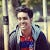# Gradient Descent in Machine Learning: Simplified

Machine learning enthusiasts have definitely heard the term “gradient descent”. It is a concept which people learn when they begin with machine learning. In this article, I have tried to explain the concept of gradient descent in a very simple and easy-to-understand manner. Finally, we will also code gradient descent from scratch.

# Top Level Idea of Gradient Descent

Gradient descent is an algorithm used for the optimization of functions, mainly used to find the local minima of a function. This algorithm is mostly used for convex functions. Why do we need gradient descent in machine learning? Well, in machine learning, to measure our model’s performance, we…

# Polynomial Curve Fitting in Machine Learning

In this article, we will attempt Polynomial Curve Fitting. The GitHub repository for the same is given at the end of the article and all the code required is included in this article as well. Polynomial curve fitting, here, is done from scratch in Python.

# INTRODUCTION

So, what is Polynomial Curve Fitting? Basically, we will try to fit a polynomial function into some custom dataset and check the results. The custom dataset, which we will create in a moment, will be non-linear and we will try to fit a 3-degree polynomial on the data. …

# Demystifying Ensemble Learning: An informal approach

In this article, I will try to demystify the concept of Ensemble Learning. People familiar with Machine Learning will have heard of a very popular algorithm called Random Forest, which is an example of ensemble learning. I will try to provide examples and with the help of them, we will build up the basics of ensemble learning, ending by discussing a few algorithms.

So, what does the word ensemble mean? According to google, ensemble is defined as a “group of items viewed as a whole rather than individually” . …

# Outliers in Machine Learning A-Z: Detection to Handling

In this article, we will go through the concept of outliers in statistics and its application in the field of Machine Learning. Starting from scratch, we will build up to identifying outliers and finally handling them.

Distribution Curves

Bell Curve

This type of curve can be considered as an idealized curve. This kind of curve has not only application in probability theory but also in many real life applications. Here, we will look at it from a statistical viewpoint. Many events such as rolling a dice, height of people, etc follow this curve. We don’t really need to know all…## Nirmalya Misra

A Machine Learning Enthusiast and a Football freak.